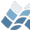NV5 GEOSPATIAL SOFTWAREFIX_GAPS

FIX_GAPS

## Purpose

This function fills in gaps in a 2D array with the average
value of its neighbors

## Calling Sequence

Result = FIX_GAPS( Data )

## Inputs

Data: A 2D array of any numeric type

## Optional Inputs

Gapsize: Set this value to 1 or 2 to specify the size of the
gaps to fill in. A value of 1 means the gap must have
2 adjacent neighbors with non Gv values. The Default
value is 1. A value of 2 means 2 adjacent gaps will
both get the average values of their neighbors. NOTE
that if gapsize=2, gaps of size 1 will still be filled
in.
Compare: Set this to the compare function used to find the
gaps. That is, compare should equal 'LT', 'LE', 'EQ',
'GE', or 'GT'. The default comparitor is 'EQ'

## Keyword Parameters

X: Set this keyword to fill in gaps in the X direction
Y: Set this keyword to fill in gaps in the Y direction
Gv: Set this keyword to the value of the gaps. This value
defaults to zero if the keyword is not set.
Edge: Set this keyword to have the algorithm wrap around the
edge of the array, and fill in gaps on the edge.

## Outputs

This procedure returns the original array, but the gaps have
been filled in by the average of its two neighbors.

## Procedure

A gap is defined as a value in the array that is 0 (zero) or
equal to the value 'Gv'. Furthermore, the gap must have two
neighbors that are *NOT* equal to Gv.
A Neighbor is defined as the array cells to the left and right
of a gap if the X keyword is set, or above and below if the Y
keyword is set.
If the Edge keyword is set, then a cell on the edge might
still have two valid neighbors.

## Example

a = indgen( 4,4 )
a[ [ 0,2,6,7,8,11,15 ] ] = 99
print, fix_gaps( data, /x, /edge, gv=99 )
print, fix_gaps( data, /y, gv=99 )

## Note

(fix_gaps(fix_gaps(d,/y),/x)) NE (fix_gaps(fix_gaps(d,/x),/y))

## Modification History

Written by: Ken Mankoff, June, 2001
Oct, 2001 Added documentation and most keywords

© 2023 NV5 Geospatial |  Legal